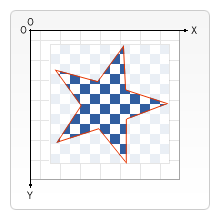# 合成效果

## `globalCompositeOperation`

`globalCompositeOperation = type`

type 字串可指定為以下 12 種合成設定之一，每一種合成設定均將套用到新繪製的圖形上。

See compositing examples (en-US) for the code of the following examples.

## 裁剪路徑裁剪路徑就像是一般畫布圖形繪圖，但就如同遮罩一樣，會蓋掉不需要的部分，如右圖所示。紅邊星星是我們的裁剪路徑，在路徑區域以外部分都不會出現在畫布上。

`clip()`

`<canvas>` 畫布預設有一個等同於本身大小的裁剪路徑，等同於無裁剪效果。

### `clip` 範例

js

``````function draw() {
var ctx = document.getElementById("canvas").getContext("2d");
ctx.fillRect(0, 0, 150, 150);
ctx.translate(75, 75);

// Create a circular clipping path
ctx.beginPath();
ctx.arc(0, 0, 60, 0, Math.PI * 2, true);
ctx.clip();

// draw background

ctx.fillRect(-75, -75, 150, 150);

// draw stars
for (var j = 1; j < 50; j++) {
ctx.save();
ctx.fillStyle = "#fff";
ctx.translate(
75 - Math.floor(Math.random() * 150),
75 - Math.floor(Math.random() * 150),
);
drawStar(ctx, Math.floor(Math.random() * 4) + 2);
ctx.restore();
}
}

function drawStar(ctx, r) {
ctx.save();
ctx.beginPath();
ctx.moveTo(r, 0);
for (var i = 0; i < 9; i++) {
ctx.rotate(Math.PI / 5);
if (i % 2 == 0) {
ctx.lineTo((r / 0.525731) * 0.200811, 0);
} else {
ctx.lineTo(r, 0);
}
}
ctx.closePath();
ctx.fill();
ctx.restore();
}
``````